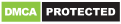# Batty Math

Batty Math is a simple but fun to play puzzle math game. It's called Batty Math because the position of the letters in the word BAT will help you solve each math puzzle. The question will use the letters B, A, & T while the answer will be numbers. To figure out the answer you'll have to remember that the digit in the hundreds digit corresponds to “B”, the tens digit to “A” and the ones digit to “T”. Touch or click the right option